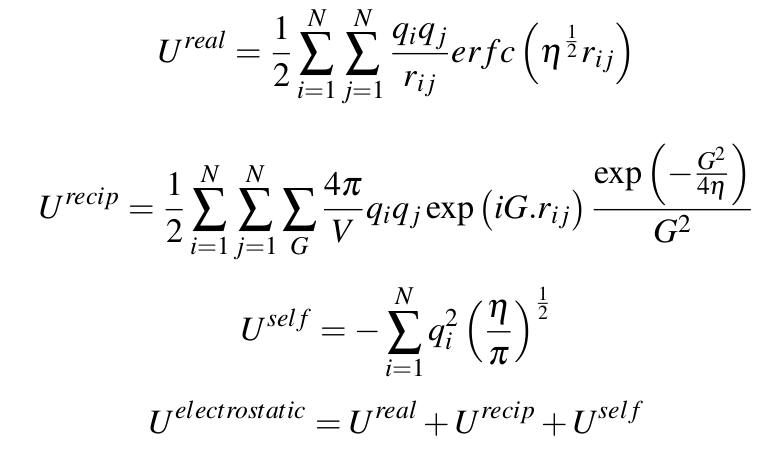# QUESTION about transfer a force filed from GULP to LAMMPS

Hi, all

thanks for the reply of steve. i did a simple test with a same molecular configuration, with only electrostatic potential. see the following output from GULP and lammps. the energy in this case differs by 0.0005 ev, (actually in the previous email, i report the energy difference between GULP and Lammps calculated on another molecular configuration is 0.05 ev, this is a typo, i am sorry for that, it should be 0.0005 ev).

LAMMPS

Memory usage per processor = 16.9445 Mbytes
---------------- Step 0 ----- CPU = 0.0000 (sec) ----------------
TotEng = -58.447991 KinEng = 0 Temp = 0
PotEng = -58.447991 E_bond = 0 E_angle = 0
E_dihed = 0 E_impro = 0 E_vdwl = 0
E_coul = -29.219132 E_long = -29.228859 Press = -43321.939
Volume = 720.52803

GULP

Components of energy :Hi, all

[...]

BY THE WAY, any one knows that if i use coul/dsf as the pair_style, what is the corresponding kspace solver i should use?

coul/dsf (like coul/wolf) is a real space only potential. thus you
should use "kspace none" (which is the default).
please recall the comment below about coul/wolf (n.b. coul/dsf is an
improved variant of coul/wolf, so what is said there applies to
coul/dsf as well):

"""
With a long enough cutoff and small enough alpha parameter, the energy
and forces calcluated by the Wolf summation method approach those of
the Ewald sum. So it is a means of getting effective long-range
interactions with a short-range potential.
"""

axel.